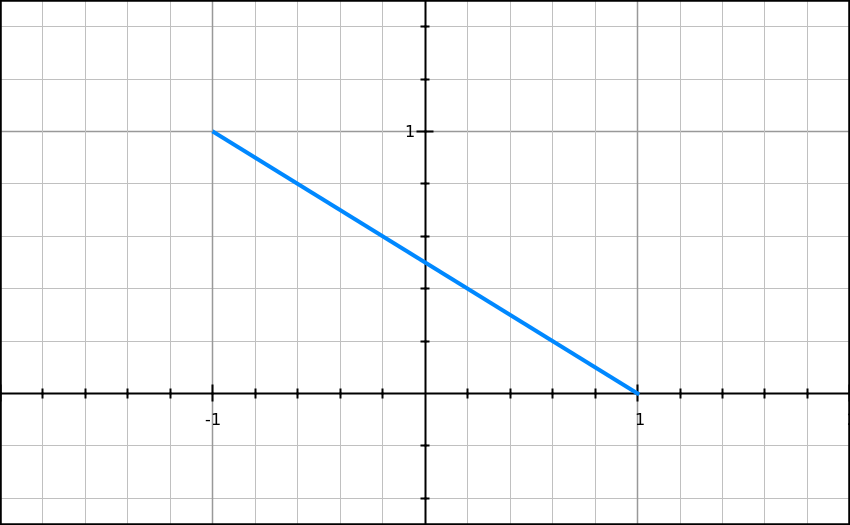## Wednesday, February 1, 2017

### Unizor - Derivatives -Problems 4

Notes to a video lecture on http://www.unizor.com

Derivatives - Problems 4

Problem 4.1
For an implicitly defined function
x=x(t),
y=y(t)
find the second derivative
y''xx(t) = y/dx².

Solution
First derivative dy/dx was discussed in the lecture on differentiation of implicitly defined function and is
y'x(t) = dy(t)/dx(t) = [dy(t)/dt]/[dx(t)/dt]
The second derivative is a derivative of the first derivative:
y''xx(t) = d/dx[y'x(t)] =
d/dx{[dy(t)/dt/ [dx(t)/dt]} =
d/dx[y't(t)/x't(t)] =
= {d/dt[y't(t)/x't(t)]}/[dx(t)/dt]=
= {d/dt[y't(t)/x't(t)]} / x't(t) =
= [y''tt(t)·x't(t)−y't(t)·x''t(t)/ {[x't(t)]²·x't(t)} =
= [y''tt(t)·x't(t)−y't(t)·x''t(t)/ [x't(t)]³ =

Problem 4.2
Using the results of Problem 4.1, find the second derivative of parametrically defined function
x=arctan(t),
y=ln(1+t²)

y''xx(t) = 2+2·t²

Problem 4.3
Using the results of Problem 4.1, find the second derivative of parametrically defined function
x=cos(2t),
y=sin²(t)

y''xx(t) = 0
This prompts that y as a function of x must be a straight line.
Indeed, since
x=cos(2t)=1−2sin²(t),
it follows that
x+2y=1 and
y = (1−x)/2.
Graph of this function is belowThe parametric definition of this function puts restrictions on its domain
−1 ≤ x ≤ 1
and range
0 ≤ y ≤ 1.
But within these boundaries y(x) is a linear function.

Problem 4.4
Using the results of Problem 4.1, find the second derivative of parametrically defined function
x=cos(2t),
y=t²